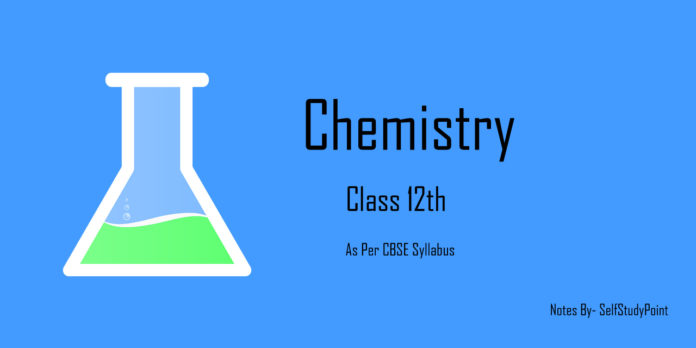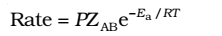According to this theory, the reactant molecules are assumed to be hard spheres and reaction is postulated to occur when molecules collide with each other.
Rate of reaction depends on the number of effective collisions which in turn depends on:

1. Energy factor: colliding molecules must have energy more than threshold energy.
2. Steric or probability factor (P): colliding molecules must have proper orientations at the time of collision.

Thus, the Arrhenius equation is modified to